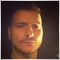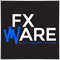# how to create five dimensional array ?237

```double array;   // this is giving me an error:
// '[' - wrong dimension

double array;      // this is working normally```28337

You cant go above 4 dimensions unfortunately237

Lorentzos Roussos:
You cant go above 4 dimensions unfortunately

thank you, but there must be a trick, like putting two multidimensional arrays together28337

Samih Abdelli:

thank you, but there must be a trick, like putting two multidimensional arrays together

You can merge the  as237

Lorentzos Roussos:

You can merge the  as 

yes, thank you, I found a way to solve my prob just with 4 dimensions array417

Maybe you can build  a 4-dimensional array of vectors. There's no standard class for vectors but in MQL5 there's CArrayDouble.

Initialisation would be as follows:

```#include <Arrays/ArrayDouble.mqh>

CArrayDouble array;

void init() {
for(int i=0;i<10;i++)
for(int j=0;j<9;j++)
for(int k=0;k<8;k++)
for(int l=0;l<7;l++) {
array[i][j][k][l].Resize(6);
array[i][j][k][l].Insert(3.14,0);
array[i][j][k][l].Insert(1.07,5);
}
}```237

lippmaje:

Maybe you can build  a 4-dimensional array of vectors. There's no standard class for vectors but in MQL5 there's CArrayDouble.

Initialisation would be as follows:

thank you but Im using MQL4485

Samih Abdelli:

thank you but Im using MQL4

Should work in MQL4 as well - ArrayDouble.mqh is also part of standard library available in MQL4.